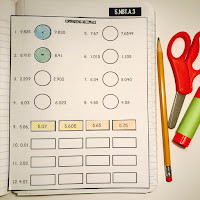## Saturday, September 23, 2017

### IMN Activities for Subtracting Decimals {Grade 5}

These interactive notebook activities are intended to help students understand how to read, write, and compare decimals to thousandths, compare two decimals to thousandths based on meanings of the digits in each place, using >, =, and < symbols to record the results of comparisons, and subtract decimals to hundredths, using concrete models or drawings and strategies based on place value, properties of operations.

Included are:
-4 different Subtracting Decimals activities to engage students
-Teacher facilitated activity for 60-90 minutes of classroom time
-Activities include: Vocabulary, Comparing and Ordering Decimals, Modeling subtracting decimals, and Practice lining up and subtracting decimals.
-Examples of Completed Interactive Math Notebook Activities are included.This set is easy to add to any Interactive Math Notebook or Math Journal for fifth graders! Teaching and reviewing subtracting decimals can be difficult, but when you break down the concepts and have the students interact with lining up the decimals then subtracting the numbers, the students will master it. Some of the best IMN activities are the ones that make the students THINK about the process and OWN their learning process. I hope your students enjoy learning with these activities too.

Happy Teaching!